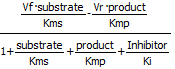# 15.2: Basic Principles of Metabolic Control Analysis (MCA)

### Introduction to Metabolic Control Analysis

Enzyme kinetics may seem difficult given the complicated mathematical derivations, the number of chemical species involved (an enzyme and all its substrates and products), the number of steps in the mechanism, and the large number of rate, kinetic, and dissociation constants. An example of such a “complicated” reaction explored earlier is shown below:But single enzymes rarely act in isolation. They are components of complex pathways which have a multitude of steps, many of which are regulated. To fully understand a reaction, it is important to study the concentrations of all species in the entire pathway as a function of time. Imagine deriving the equations and determining all the relevant concentrations and constants of a pathway such as glycolysis!

To study enzyme kinetics in the lab, you have to spend much time in developing assays to measure how the concentration of species changes as a function of time to be able to measure the initial velocities of an enzyme-catalyzed reaction. However, in networks of connected metabolic reactions, the concentration of some species in the system may not change. How can this happen? Two simple examples might help explain how.

Example 1: There is zero input or output from a given reaction. This would occur in a closed system for a reversible reaction at equilibrium.

For a reversible reaction of reactant R going to product P with forward and reverse first order rate constants, the following equation can be written at equilibrium:At equilibrium, R and P don’t change.

Example 2:  Consider the reaction as part of a pathway of reactions (like an open system). Now imagine a nonzero input to form reactant R and a nonzero output that consumes product P. If the input and output rates are the same, the concentrations of R and P would not change with time. That is the rate of formation of a reactant R for a given reaction is equal to the rate at which the product P of the give reaction is used. This would lead to a steady state but not equilibrium concentration of the species.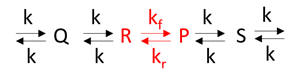Understanding a pure enzyme in vitro and in vivo requires different approaches. Biochemists like to isolate and purify to homogeneity an enzyme found in some tissue and study its mechanism of action. In doing thermodynamic measurements to measure equilibrium constants (Keq) or dissociation constants (KD), from which ΔG0 can be calculated, a protein concentration is usually held constant as the binding ligand concentration is varied (independent variable). A dependent variable signal (often spectroscopic) is measured. Measurements are made when equilibrium is reached.

For enzyme kinetic measurements in vitro, the enzyme concentration is usually held constant while substrate and modifiers are varied (independent variables) to determine how velocity (dependent variable) changes. The velocity is determined by the substrate concentration. When inhibition is studied, the substrate is varied while the inhibitor is held constant at several different fixed concentrations.

In vivo, the substrate concentration and even the enzyme concentration are determined by the velocity. Again compare this to in vitro kinetics when concentrations determine the velocity
For sets of reactions in pathways, it is better to use the term flux, J. In the steady state, the in and out fluxes for a given reaction are identical. Flux J is used to describe the rate of the system whereas rate or velocity v to used describe the rate of an individual enzyme in a system.

Computer programs can find steady states concentrations by finding the roots of the ordinary differential equations (ODE) when set to zero (vf = vr). To model a process at very low concentrations, programs can also use probabilistic or stochastic simulations to model probability distributions for species and their change with time for a finite number of particles. In such simulations, concentrations (mM) are placed with number of particles. ODEs don’t work well to describe these conditions since changes in concentrations are not continuous.

Now back to our earlier rhetorical question of deriving the equations and determining all the relevant concentrations and constants of a pathway such as glycolysis! It actually has been done by Teusink et al for glycolysis in yeast. In fact, many such complicated metabolic and signal transduction pathways have been mathematically modeled in the hopes of better understanding cellular and organismal responses. Quantitatively modeling and predicting input, outputs, and concentrations of all species in complex pathways is the basis of systems biology.

### Basic Principles of Metabolic Control Analysis

A variety of inputs are required for such computational analyses:

a. Defined pathways. These are available in many databases. An examples from KEGG pathways for yeast glycolysis is shown belowhttp://www.genome.jp/dbget-bin/www_bget?pathway:sce00010

b. A computational modeling program to input all parameters, equations, models and calculate concentrations for all species as a function of time. Free, downloadable programs that do that include COPASIVirtual Cell, and Cell Designer. An example of a mapped model from Cell Designer for yeast glycolysis with several additional reactions branching off from the classical glycolytic pathway is shown below.The computational results from the analyzes for yeast glycolysis were able to fit experimental data only if corrected by addition of several branching reactions (shown in the figures above and below):c. A list of all species involved in the pathway (shown in the figure below for glycolysis and branching reactions).d. A list of all reactions (shown below for glycolysis and branching reactions, taken from COPASI)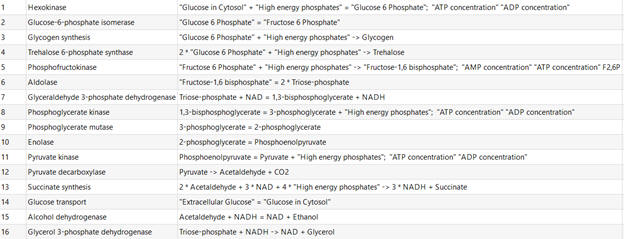e. Parameters of all species. An example is shown below for hexokinase (taken from COPASI).f. Equations that can be used to compute the change in concentrations of all species with time. These are usually ordinary differential equations (ODE) as described in Chapter 6B - Kinetics of Simple and Enzyme-Catalyzed Reactions, Sections B1: Single Step Reactions.) ODEs are easy to write but require a computer to solve as the number of interacting species increases.

An example chemical reaction and a set of ODEs for each species is shown below.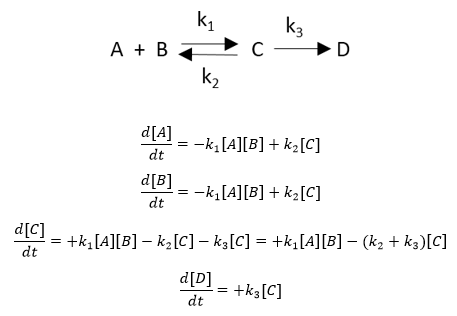If a reaction removes species X, the right side of the ODE for the disappearance of that species has a – sign for that term. Likewise, if a reaction increases species X, the right hand side has a + sign. The above examples show unimolecular (C to D, C to A + B) and bimolecular (A+B to C) reactions.

An example of an ODE taken from COPASI is shown below for the change in concentration of glucose-6-phosphate.Databases containing curated models with all above information have been developed for many pathways. The yeast glycolysis model described above is found in the Biomodels Database. The model, BIOMD0000000064, can be downloaded as a systems biology markup language (SBML) file and imported into any of the programs described above.
The graphs below show the concentrations for select and all species as a function of time for yeast glycolysis using COPASI.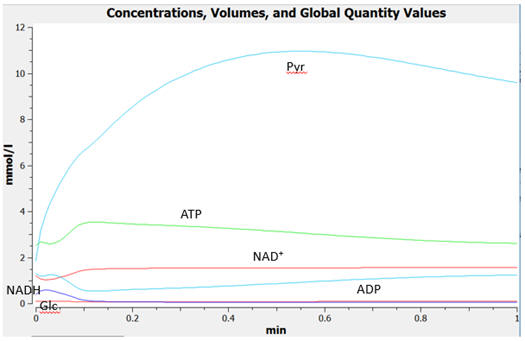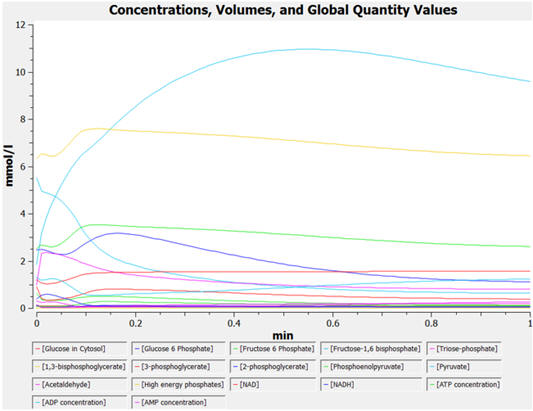### Metabolic Control Analysis and Simple Enzyme Inhibition

Biochemists model complex enzyme-catalyzed reactions in the presence and absence of modifiers (either activators or inhibitors) to develop mechanisms for the reactions. The following kinetic parameters are experimentally determined by fitting initial velocity (vo) vs substrate concentration ([S]) through nonlinear fitting algorithms.

• Km – the Michaelis Constant, the concentration of substrate at half-maximal velocity;
• Vm – the velocity at saturating substrate concentration;
• kcat - the turnover number for conversion of bound reactant to product;
• Kix – inhibition dissociation constants.

An example of competitive inhibition illustrates a common type of analysis for such reactions.A modern pictorial depiction of a simple irreversible, enzyme-catalyzed reaction of substrate S going to product P with inhibition by the product and by an added inhibitor is shown below: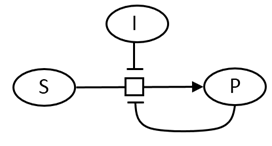Consider the simple enzyme-catalyzed reaction for a reversible conversion of substrate S to product P that has 3 reversible steps.If the forward (f) and reverse (r) chemical reaction steps (reactions 2 and -2) were irreversible and written separately, simple Michaelis-Menten equations could be written for eachFor the actual reversible reactions for step 2, the net forward rate cannot be found by simple subtraction of the two equations above as the differential equations describing the simple forward and reverse rates don’t account for the reverse steps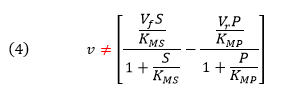A simple derivation (assuming rapid equilibrium for both forward and reverse steps) can be made for the net forward reaction. Again consider the following enzyme catalyzed reaction:You may remember that for the isolated E + S <----> ES and for E + P <----> EP reactions, the simple dissociation constants, KS and KP are given byThe rapid equilibrium assumption states that the rate of dissociation of ES and EP, which are both physical steps, are much faster than the rate of the chemical conversion steps for each complex. Hence k-1 >> k2 and k3 >> k-2, so the relative amounts of ES and EP can be determined from the dissociation constants as shown above.

Mass conservation of enzyme givesFrom this we can get the fractional amount of both ES and EPNow we can derive the rate equation for the net forward reaction for the rapid equilibrium case: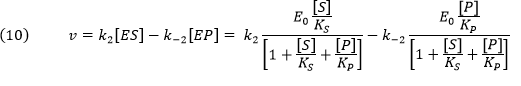Knowing that k2E0 and k-2E0 represent the maximal velocities, Vf and Vr, respectively, the equation becomes:An equation of similar form can be derived from the steady state assumption. This equation is clearly different from the earlier equation (4) derived by intuitively assuming a simple subtraction of the irreversible forward and reverse rates which we have now shown to be invalid by comparison.Programs like COPASI have many built in equations for velocities of many enzyme-catalyzed reactions that have similar forms. Two are shown below:

Reversible Michaelis-Menten:Competitive Inhibition Reversible: